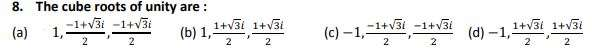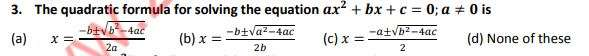# FSC Mathematics MCQs Chapter 4

FSC Math MCQs NUST entry test 2023 NET contains most of the Mathematics MCQs. FSC Part 1 Math book MCQs. HSSC Mathematics questions MCQs. Chapterwise mathematics MCQs for first year 11 class MCQs.

673
Created on

1 / 20

The sum of all four fourth roots of unity is:

2 / 20

The complex fourth roots of unity are ……. of each other.

3 / 20

4 / 205 / 20

Basic techniques for solving quadratic equations are

6 / 20

The complex cube roots of unity are………………. each other.

7 / 208 / 20

The product of all four fourth roots of unity is:

9 / 2010 / 20

Synthetic division is a process of:

11 / 20

The equations involving redical expressions of the variable are called:

12 / 20

If product of all cube roots of unity is equal to ?^? + ? , then ? is equal to:

13 / 20

The equation in which variable occurs in exponent , called:

14 / 20

If 2 and -5 are roots of a quadratic equation , then equation is:

15 / 20

The sum of all four fourth roots of 16 is:

16 / 2017 / 20

Product of cube roots of -1 is:

18 / 20

Sum of all cube roots of 64 is :

19 / 20

The complex cube roots of unity are………………. each other.

20 / 20

The average score is 57%

0%

### 2 responses to “FSC Mathematics MCQs Chapter 4”

1. Anonymous says:

W=_____
a,-w²
b,w-²
c,1+w²
d, both a and b

• Anonymous says:

B because through the product rule 1x W xW^2 = 1

Salam